# Equation - math word problems - page 47

1. Car driver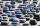The car driver is in town A and is scheduled to be in town B at a specified hour. If it travels at an average speed of 50km/h, it will arrive in city B 30 minutes later. But if he travels at an average speed of 70km/h, he would arrive half an hour earlier
2. The train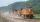The train passed the observer in the 20s and passed through a 1500m tunnel in 2 minutes. Calculate the speed (km/h) and train length.
3. Three paintersOne painter would have painted the fence for 15 hours, the second in 12 hours. The plot had to be painted in four hours, so they called the third one, and all worked together. For what time would the third painter paint fence the fence alone?
4. My fatherMy father has a big farm. 6/8 of it were planted with mango trees, 1/2 of the remainder are guava trees and the rest 10 trees are santol trees. What is the number of all trees?
5. Two pipesHow long will the pool be filled with a double supply pipe if it takes the pool to fill the first pipe by 4 hours longer and the second pipe 9 hours longer than both pipes open at the same time?
6. PeroxideHow many ml 30% of peroxide (H2O2) should be poured into 100ml H2O to give a 20% solution?
7. SeamstressThe seamstress cut the fabric into 3 parts. The first part was the eighth fabric, the second part was three-fifths of the fabric and the third part had a length of 66 cm. Calculate the original length of the fabric.
8. OrchardOne-eighth of the trees in the fruit plant in winter froze and one-twelfth of damaged disease and pests. Healthy trees remained 152. Is it enough to supply 35 trees to restore the original number of trees in the orchard?
9. Average ageThe company of five people has an average age of 46 years. The average age of the first four is 43 years. How many years has the fifth member of this company?
10. Sale discountThe product was discounted so that eight products at a new price cost just as five products at an old price. How many percents is the new price lower than the old price?
11. Our houseOur house would paint four painters in 14 days. How long could it paint five painters? How long will it take if two other painters will come to help after 5 days?
12. Birthday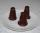Mother bought 21 desserts on the occasion of Mirka's birthday one tips was 9 CZK and the kremlin cost 12 CZK. For all desserts, she paid 213 CZK. How many kremlins and how many tips mums did buy?
13. Pupil ageI am a pupil of the primary school, I spent 1/4 of my age on the exercise of parents with children, 1/3 on drawing, 1/6 on flute. I did not have a ring for the first three years of my life and never went to two rings at once. How old am I?
14. Exponential equationDetermine the value of having y in the expression (3^y): (4^-1)=36.Y is a natural number greater than zero.
15. Two diggersTwo diggers should dig a ditch. If each of them worked just one-third of the time that the other digger needs, they'd dig up a 13/18 ditch together. Find the ratio of the performance of this two diggers.
16. Harvesters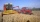The first harvester will harvest the grain from the field in 20 hours, the second, the more powerful in 10 hours. How long will take harvest this field by both harvesters, if the second harvester has to be set up first and this takes 2 hours? The first har
17. Eggs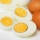3400 eggs sliced hens for February and March. We have to calculate how many hens can make 3400 eggs when one hen give 2 eggs a day for 59 days.
18. Simple equationSolve the following simple equation: 2. (4x + 3) = 2-5. (1-x)
19. FractionsThree-quarters of an unknown number are 4/5. What is 5/6 of this unknown number?
20. Four numbersThe first number is 50% second, the second number is 40% third, the third number is 20% of the fourth. The sum is 396. What are the numbers?

Do you have an interesting mathematical word problem that you can't solve it? Enter it, and we can try to solve it.

To this e-mail address, we will reply solution; solved examples are also published here. Please enter the e-mail correctly and check whether you don't have a full mailbox.

Please do not submit problems from current active competitions such as Mathematical Olympiad, correspondence seminars etc...

Do you have a linear equation or system of equations and looking for its solution? Or do you have quadratic equation?